FUNCTIONAL
DIFFERENTIAL
EQUATIONS VOLUME 4
1997, NO 1-2
PP. 3 - 11 MATHEMATHICAL WORK OF VLADIMIR MAZ'YA
(ON THE OCCASION OF HIS 60th BIRTHDAY)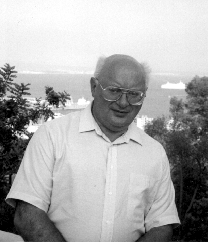V. Maz'ya

In 1990 Vladimir Maz'ya and his family emigrated to Sweden. Now he is a Professor at the Linköping University.

Vladimir Maz'ya is an outstanding mathematician of excellent international reputation. The results of his profound and extensive researches were published in more than 300 papers and several books.

V. Maz'ya made a valuable contribution to the Sobolev spaces theory, theory of capacities, qualitative and asymptotic theories of linear and nonlinear elliptic equations, theory of multipliers in spaces of differentiable functions, approximation theory as well as to the development of the boundary integral equations method, to the study of the degenerate oblique derivative problem, the Cauchy problem for elliptic and hyperbolic equations, the maximum principle for elliptic and parabolic systems, boundary value problems in domains with piecewise smooth boundaries etc. Of special notice is that in 1968 he succeeded in constructing first counterexamples for the 19th and 20th Hilbert problems for higher order elliptic equations and regular variational problems.

It should be particularly emphasized that being originally a "pure" mathematician, V. Maz'ya also displayed his keen interest in mechanics of continua. In particular, in 1977 he solved a problem of oscillations of fluid in the presence of a submerged body, stated by F. John in 1950. In the field of hydrodynamics he was dealing with three types of problems: mathematical theory of surface waves, Navier-Stokes equations in domains with irregular boundaries, and the three-dimensional problem of steady-state motion of a fluid with a free surface. A considerable part of his activities is connected with elasticity; he worked on the Cosserat spectrum, dynamics of stresses under fast heat processes, the polygon-circle paradox in the thin plate theory, an algorithm for the solution of the Lamé system with data on a part of the boundary.

Although the comprehensive account of V. Maz'ya's scientific activities is hardly possible in the framework of a short article, we hope that the following outline can provide a clear idea regarding the importance, depth and diversity of V. Maz'ya's achievements during the period 1960-1997.

1. Equivalence of isoperimetric and integral inequalities.

Sobolev spaces

In 1960, being a student of the Leningrad University, V. Maz'ya found necessary and sufficient conditions for the validity of certain imbedding theorems for the Sobolev space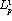which characterize domains and weight functions. For p=1 these conditions are formulated in terms of "isoperimetric inequalities" connecting the volume and the area of the part of the boundary of an arbitrary subset of the domain. The equivalence of geometric isoperimetric inequalities and integral inequalities of the Sobolev type proved to be extremely fruitful and was explored in many subsequent papers. He also showed that for p>1, geometric set functions, such as volume and area, are insufficient for an adequate description of the properties of domains, and that here the isoperimetric inequalities between the volume and the so-called p-capacity arise. Such criteria enabled V. Maz'ya to obtain necessary and sufficient conditions for various spectral properties of the Schrödinger operator (1962, 1964).

The above mentioned results, stated in terms of isoperimetric inequalities, are closely connected with the so-called strong type capacitary inequalities discovered by V. Maz'ya (1972).

Since 1960 V. Maz'ya started his spectacular study of various aspects of the theory of Sobolev spaces. His pioneering results influenced the subsequent development of the theory of Sobolev spaces in a crucial way. His earlier works were summarized in his famous book "Sobolev spaces" (Springer-Verlag, 1985). The new book "Differentiable functions on bad domains" by V. Maz'ya and S. Poborchi (World Scientific Publishers, 1997) reflects the recent results of the authors in this field.

2. Theory of capacities and nonlinear potentials

In 1963 V. Maz'ya introduced the so-called polyharmonic capacity and used it to obtain necessary and sufficient conditions on the domain ensuring the unique solvability of the Dirichlet problem in the energy space and the discreteness of the spectrum of the corresponding operator. In 1970 V. Maz'ya and V. Havin introduced nonlinear potentials and investigated their basic properties. Nowadays it became a vast area of study which gives answers to many important questions in function theory.

3. Counterexamples related to the 19th and 20th Hilbert

problems

According to Hilbert's hypothesis, solutions of second order quasilinear elliptic equations with analytic coefficients and the first order regular analytic variational problems are smooth and even analytic. By the end of the sixties this had been justified in full generality and it was believed that the same is true for equations and variational problems with higher derivatives. All the more surprising became V. Maz'ya's examples of non-smooth solutions of higher order quasilinear elliptic equations with analytic coefficients and regular analytic variational problems (1968). In the same paper, simultaneously with and independently of E. De Giorgi, V. Maz'ya gave examples of unbounded solutions to linear higher order divergence elliptic equations, thus showing that the classical Nash-De Giorgi regularity theorem is no more valid for such equations.

4. Boundary behaviour of solutions to elliptic equations

From his early work and until now V. Maz'ya has been interested in the behaviour of solutions to partial differential equations near the boundary. He used isoperimetric inequalities to obtain precise conditions on the boundary ensuring various estimates of solutions to the Dirichlet and Neumann problem for the second order elliptic equations with bounded measurable coefficients. In his article of 1962 he found a certain estimate for the continuity modulus of a harmonic function formulated in terms of the Wiener integral. This estimate and its extensions, obtained by V. Maz'ya and many others, became an important tool in the qualitative theory of linear and nonlinear elliptic equations.

His short article of 1977 contains a necessary and sufficient condition for the coincidence of the Martin and Euclidean topologies for harmonic functions on domains which are solids of revolution whose boundaries consist of two tangent components.

The same year V.Maz'ya found a sufficient condition for the Wiener regularity of a boundary point with respect to a quasilinear elliptic equation of a certain type. To prove the necessity of V. Maz'ya's condition remained an open problem for a long time which was solved only recently by T. Kilpeläinen and J. Malý (1994). All existing results about the Wiener regularity of a boundary point with respect to higher order linear elliptic equations were obtained by V. Maz'ya either alone or together with his collaborators. In particular, in 1984 he and S. Nazarov found an example of a strongly elliptic fourth order equation with constant coefficients for which the vertex of a cone is not regular in the Wiener sense.

5. Non-elliptic singular integral and pseudodifferential

operators. Degenerating oblique derivative problem

In 1965, influenced by S. Mikhlin, V. Maz'ya and B. Plamenevskii studied well-posed problems for singular integral equations with symbol vanishing on a finite number of cones. Those were the first works on non-elliptic multidimensional pseudodifferential operators.

In the late sixties, V. Maz'ya and B. Paneah began a thorough study of degenerate elliptic pseudodifferential operators on smooth manifolds without boundary. They introduced a classification of the types of degeneration and developed a solvability theory for each of them. Moreover, they obtained precise results on the regularity of weak solutions.

The classic oblique derivative problem is an important example of the class of operators investigated by Maz'ya and Paneah, provided the vector field is tangent to the boundary along a submanifold of codimension 1 and transversal to this submanifold. Not long before this geometrical situation was directly studied by Yu. Egorov and V. kondratiev and M. Malyutov, who used different methods.

In 1972 V. Maz'ya published a deep result on the unique solvability of the oblique derivative problem without the aforementioned transversality condition. Until present, this is the only known result in the case of the general type of degeneration (in V. Arnold's sense).

6. Estimates for general differential operators

Necessary and sufficient conditions ensuring various estimates for solutions of differential equations in the half-space were found by V. Maz'ya and I. Gel'man. These results were summarized in their book "Abschätzungen für Differentialoperatoren in Halbraum", 1982.

7. The method of boundary integral equations

In the sixties Yu. Burago and V. Maz'ya, inspired by a question of F. Riesz and B. Sz.-Nagy, developed a theory of simple and double layer harmonic potentials in spaces C and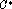under minimal assumptions on the boundaries of domains. V. Maz'ya and G. Kresin found explicit expressions for the essential norm of the double layer elastic and hydrodynamic potentials in the space of continuous vector-functions (1979, 1995). As a by-product they proved that, unexpectedly (in contrast to the double layer harmonic potential), the essential norm of a boundary integral operator of the plane elasticity theory is greater than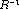where R is the Fredholm radius of the same operator.

In 1981 V. Maz'ya proposed a new method for the study of boundary integral equations in domains with piecewise smooth boundaries based upon preliminary investigation of the properties of solutions of some auxiliary boundary value problems. This method enabled him and his colleagues to obtain complete results on solvability in the theory of harmonic and elastic potentials on surfaces with polyhedral angles, edges and cusps as well as on asymptotic representations of the solutions of integral equations of potential theory near singularities of the boundary. As an application of the same method, V. Maz'ya and his student N. Grachev gave an affirmative answer to the classical question on the solvability of the integral equation of the Dirichlet problem in the space C for an arbitrary polyhedron. At the same time, this problem was solved by A. Rathsfeld by a different method. A theory of boundary integral equations on plane contours with cusps, developed by V. Maz'ya and A. Soloviev during the last decade, is the only progress in this domain.

8. Linear theory of surface waves

In two papers of 1973 V. Maz'ya and B. Vainberg studied basic boundary value problems of the linear theory of surface waves. The main difficulty here was to prove the absence of eigenvalues on the continuous spectrum of some boundary operator. Earlier, F. John, when studying the stationary problem on steady-state oscillations of a fluid layer in presence of a semi-immersed body, put the problem to find solvability conditions in the case of immersed body. The problem was solved by V. Maz'ya in 1977. In the eigthies he and N. Kuznetsov developed an asymptotic approach to non-stationary problems of the linear theory of surface waves under short-term or fast oscillating perturbations. In 1997 the first solvability theorem to the problem of the motion of a submersed circular cylinder for an arbitrary Froude number was published by V. Maz'ya and M. Livshits. In a paper of 1993 V. Maz'ya and B. Vainberg obtained an asymptotic representation at infinity of Green's function for the linear problem on the uniform motion of a source in a fluid. The advantage of this representation is that it is uniform with respect to all directions and depths.

9. The Cauchy problem for the Laplace equation

In 1974, together with V. Havin, V. Maz'ya found the best possible conditions for uniqueness of harmonic functions with the prescribed behaviour of the gradient at a boundary point.

10. Theory of multipliers in spaces of differentiable functions

In 1979-1983, together with T. Shaposhnikova, V. Maz'ya developed a theory of multipliers in spaces of differentiable functions. They gave a description of various classes of multipliers and found deep applications to boundary value problems and function theory. These results were summarized in their book "Theory of multipliers in spaces of differentiable functions", Pitman, 1985.

11. Characteristic Cauchy problem for hyperbolic equations

Together with B. Vainberg, V. Maz'ya proved the unique solvability of the Cauchy problem for general hyperbolic equations with data on a surface an arbitrary part of which is characteristic (1981).

12. Boundary value problems in domains with piecewise

smooth boundaries

As mentioned in Sec. 4, V. Maz'ya began working on elliptic boundary value problems in domains with nonsmooth boundaries in the sixties. Initially he concentrated on second order equations. For example, while studying conditions of the selfadjointness of the Laplacian with zero boundary conditions for plane domains of classbut not of class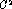, he arrived at the striking effect of instability of the index under affine transformations of coordinates.

After appearance of V. Kondratiev's fundamental paper on general elliptic boundary value problems in domains with conic singularities V. Maz'ya began his active work in this field together with B. Plamenevskii, and later with J. Rossmann and V. Kozlov. As a result, a vast theory was developed including a priori estimates for norms in various spaces, estimates for fundamental solutions, asymptotic representations for solutions etc. In particular, V. Maz'ya and B. Plamenevskii proposed a method for calculation of coefficients in the asymptotics of solutions to boundary value problems near singularities of the boundary. A substantial monograph by V. Kozlov, V. Maz'ya and J. Rossmann on the elliptic theory for domain with point singularities of the boundary has recently been published by the American Mathematical Society.

13. Asymptotic theory of differential and difference

equations with operator coefficients

Together with B. Plamenevskii and later with V. Kozlov, V. Maz'ya developed an asymptotic theory of ordinary differential equations with strongly perturbed unbounded operator coefficients. V. Maz'ya and M. Sulimov described the asymptotic behaviour of solutions of difference equations with operator coefficients in Hilbert spaces.

14. Maximum modulus principle for elliptic and

parabolic systems

In a series of joint papers, V.Maz'ya and G. Kresin gave an algebraic characterization of strongly elliptic and parabolic differential systems of second order satisfying the maximum modulus principle. V. Maz'ya and J. Rossmann showed that the Miranda-Agmon maximum principle holds for any strongly elliptic operator in an arbitrary plane domain bounded by a piecewise smooth contour without zero angles. A similar result was obtained in 3 dimensions and it was proved that in the casesome additional geometric requirements on the boundary are needed.

15. Iterative procedures for solving ill-posed boundary

value problems

New iterative procedures were proposed by V. Kozlov and V. Maz'ya for ill-posed boundary value problems, in which every step is reduced to the solution of well-posed problems for the original differential equation. The regularizing character of the algorithms is ensured only by an appropriate choice of boundary conditions for each iteration.

16. Asymptotic theory of singularly perturbed boundary

value problems

The book "Asymptotische Theorie elliptischer Randwertaufgaben", vol. 1 and 2, 1991 by V. Maz'ya, S. Nazarov, B. Plamenevskii is dedicated to the construction of asymptotic series for solutions of elliptic boundary value problems under singular perturbations of domains (blunted angles, conic points or edges, small holes, narrow passages etc.). Here a new general approach to problems of such a kind is proposed.

A new progress in the same area was achieved by V. Maz'ya in collaboration with V. Kozlov and A. Movchan. They solved the problem of asymptotic analysis of junctions of 3-dimensional and 1-dimensional components of elastic multistructures. These results are collected in their joint monograph "Fields in multistructures. Asymptotic analysis" (to appear in Oxford University Press).

17. "Approximate approximations" and their applications

In 1991 V. Maz'ya proposed a new approximation method mainly directed to the numerical solution of operator equations. This method is characterized by a very accurate approximation in a certain range relevant for numerical computations, but in general the approximations do not converge. The main applications concern the evaluation of multidimensional volume potentials and semianalytic time-marching algorithms for solving nonlinear evolution equations. The method is especially useful when applied to nonlinear evolution equations with non-local operators, where finite element and finite difference schemes are not directly applicable. This field is being exploped by V. Maz'ya and V. Karlin.

V. Maz'ya and G. Schmidt developed an extensive theory of such approximations. Moreover, they introduced the so-called approximate wavelet decompositions of spaces of approximating functions which appeared to be very useful for constructing high order semi-analytic cubature formulas for important classes of pseudodifferential and other integral operators of mathematical physics (1996).

Finally it is worth mentioning V. Maz'ya's active interest in the history of mathematics. A substantial book "Jacques Hadamard, a Universal Mathematician" written in collaboration with T. Shaposhnikova is to be published soon by the American and London Mathematical Societies.

The presented concise classification of V. Maz'ya's scientific activities is incomplete not only because the printing space is limited. In fact, any attempt to reach a certain comprehensiveness is doomed to failure since Vladimir Maz'ya, with his inexhaustible energy, will undoubtedly obtain new remarkable mathematical results during the time between the writing and publication of the present article.

We wish to V. Maz'ya, our friend and colleague, happy birthday, plenty of health and continuation of new and profound research. All of us who have been privileged to know and to work with him have the deepest admiration and affection for him.

D. Eidus, A. Khvoles, G. Kresin, E. Merzbach,

S. Prössdorf, T. Shaposhnikova, P. Sobolevskii, M. Solomiak